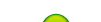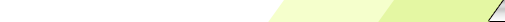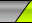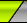Friday, 2020-02-28BioInfo PakistanHome | Sign Up | Log InWelcome Guest | RSS
Section categories
 Related Subjects This category includes brief overview of all related subjects. Defining BioInformatics In this section we tried to briefly explain what bioinformatics is ? Unviersities This contains information about universities that are offering bioinformatics degree programs. Resources Contains information about bioinformatics resources including databases, tools and techniques. Algorithms This category includes some of the basic algorithms that are usually used by bioinformaticians.
 Our poll Pakistani Students Should Join Bio-Informatics Yes No Total of answers: 35
 Chat Box Only authorized users can post messages
 StatisticsTotal online: 1 Guests: 1 Users: 0
Home » 2011 » August » 15 » Hirschberg's Algorithm Pseudocode 2
2:39 PM
Hirschberg's Algorithm Pseudocode 2

 Hirschberg's Algorithm Pseudocode1. Pseudocode:High level description of Hirschberg's algorithm:`Hirschberg(x,y) is ````1. n = length(x); m = length(y) ``````2. If n <= 1 or m <= 1: `````` OUTPUT Alignment(x,y) using Needleman-Wunsch. `````` Else: `````` A. mid = floor(n/2) `````` B. x_left = Prefix[x,mid] `````` C. x_right = Suffix[x,n-mid] ```` D. Edit[x_left] = Forwards(x_left,y)```` %% an array of length m+1 ```` E. Edit[x_right] = Backwards(x_right,y)```` %% an array of length m+1 ```` F. cut = ArgMin{Edit[x_left,Prefix[y,j]] +```` Edit[x_right,Suffix[y,m-j]]} %% j ranges from 1 to m-1 `````` G. Hirschberg(x_left,Prefix[y,cut]) ```` H. Hirschberg(x_right,Suffix[y,m-cut])`Page 1
Category: Algorithms | Views: 1660 | Added by: Ansari | Rating: 0.0/0